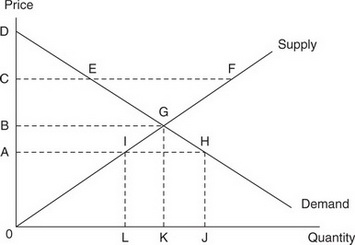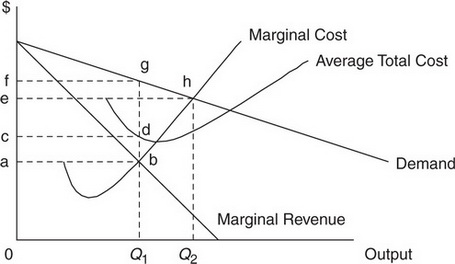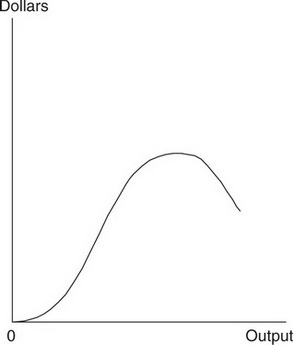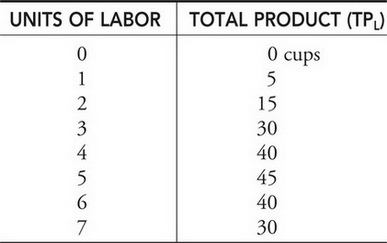# AP Microeconomics Practice Test 20

### Test Information12 questions14 minutes

1.If the current price is 0B, we would expect

2.If the price were to fall from 0C to 0A, which of the following would be true?

3.If the market is in equilibrium, which of the following areas corresponds to producer surplus?

4. The downward-sloping demand curve is partially explained by which of the following?

5. Dorothy has daily income of \$20, each cup of coffee costs Pc = \$1, and each scone costs Ps = \$4. The table below provides us with Dorothy's marginal utility (MU) received in the consumption of each good. As a utility-maximizing consumer, which combination of coffee and scones should Dorothy consume each day?6. You are told that the Gini coefficient of income inequality has risen from .35 to .85. Which of the following is a likely cause of this change?

7.The figure above best represents which of the following functions?

8. If it is true that bacon and eggs are complementary goods, then

9. A firm employs variable amounts of labor to a fixed amount of capital to produce output. If the daily wage paid to labor increases, how does this affect the firm's costs?

TOTAL VARIABLE COST     TOTAL FIXED COST     TOTAL COST

10. Diminishing marginal returns to short-run production begin when

11. Which of the following is a characteristic of perfect competition?

12.The table above shows how hiring increasing amounts of labor to a fixed amount of capital affects the hourly output of Eli's lemonade stand. Based on this table of production data, which of the following can be said?